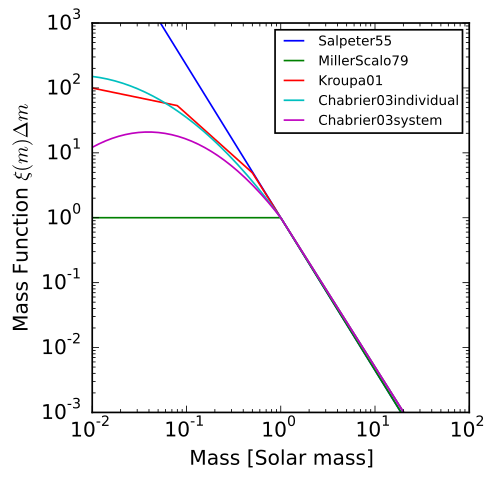# IMF

Initial mass function##### Initial mass function

empirical function that describes the initial distribution of masses for a population of stars

In astronomy, the initial mass function (IMF) is an empirical function that describes the initial distribution of masses for a population of stars. The IMF is an output of the process of star formation. The IMF is often given as a probability distribution function (PDF) for the mass at which a star enters the main sequence. The distribution function can then be used to construct the mass distribution of a population of stars. It differs from the present day mass function (PDMF), the current distribution of masses of stars, due to the evolution and death of stars which occurs at different rates for different masses as well as dynamical mixing in some populations.